﻿ 非稳定动态过程非定常气动力建模Download PDF文章快速检索 高级检索

Unsteady aerodynamic modeling of unstable dynamic process
CHEN Senlin, GAO Zhenghong, ZHU Xinqi, PANG Chao, DU Yiming, CHEN Shusheng
School of Aeronautics, Northwestern Polytechnical University, Xi'an 710072, China
Abstract: Current unsteady aerodynamic modeling methods at high angle of attack usually use stable vibration test data at multiple frequencies to predict stable hysteresis loop. However, the rapid maneuvering process of aircraft, such as post-stall maneuver, cannot be a constant and stable vibration, but an unstable dynamic process. Therefore, the aerodynamics would not reach a stable hysteresis loop, but would always be in the initial unstable process of entering the hysteresis loop. The vibration theory analysis shows that the dynamic response process of unstable aerodynamics has the unstable and stable stages. The traditional modeling method focuses on the stable stage, while the actual maneuvering process of aircraft is in the unstable stage. Based on the Least Squares Support Vector Machine (LS-SVM), an excitation input suitable for nonlinear system identification is introduced to model unsteady aerodynamic forces of any motion in the amplitude and frequency ranges at high angle of attack. After completing the model training, the method is applied to predict the lift coefficient, drag coefficient, and pitching moment coefficient of a wing at high angle of attack with different reference states in pitching motion. The results show that not only the stable hysteresis loop is accurately predicted, but also the initial unstable process of entering the hysteresis loop is accurately predicted. In addition, the results also show that the reference state has significant influence on the characteristics of aerodynamics in the initial process. Further validation also shows that modeling based on the stable hysteresis loop data can only predict the stable hysteresis loop, and cannot predict the unstable process of entering the hysteresis loop.
Keywords: unsteady aerodynamics    high angle of attack    unstable dynamic process    least squares support vector machine    nonlinear system    system identification    input design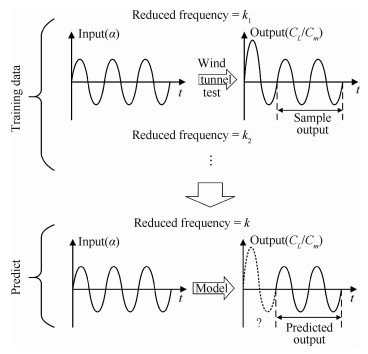图 1 基于风洞试验数据的气动力建模方法示意图 Fig. 1 Schematic diagram of aerodynamic modeling method based on wind tunnel test data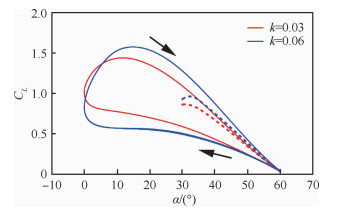图 2 某机翼做俯仰运动时的升力系数滞环(Ma=0.4, Re=3.0×106) Fig. 2 Lift coefficient hysteresis of a wing for pitching motion at Ma=0.4 and Re=3.0×106

1 激励模型 1.1 非稳定动态过程和非线性系统辨识

 $\ddot y + 2\zeta p\dot y + {p^2}y = {Y_0}{p^2}{\rm{cos}}(\omega t)$ （1）

 ${y = {y_1} + {y_2}}$ （2）
 ${{y_1} = {{\rm{e}}^{ - \zeta pt}}(A{\rm{sin}}(qt) + B{\rm{cos}}(qt))}$ （3）
 ${{y_2} = Y{\rm{cos}}(\omega t - \varphi )}$ （4）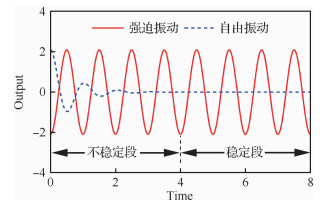图 3 某振荡器在正弦激励下的强迫振动响应和自由振动响应 Fig. 3 Forced vibration response and free vibration response of an oscillator under sinusoidal excitation

1.2 激励的选择和设计

 $\mathit{\boldsymbol{u}} = \sum\limits_{i = 1}^M {{A_i}} {\rm{sin}}\left( {\frac{{2\pi it}}{T} + {\varphi _i}} \right)$ （5）

 ${\rm{RMS}} (\mathit{\boldsymbol{u}}) = \sqrt {\frac{{{\mathit{\boldsymbol{u}}^{\rm{T}}}\mathit{\boldsymbol{u}}}}{N}}$ （6）

 ${\rm{RPF}} (\mathit{\boldsymbol{u}}) = \frac{{{\rm{max}}(\mathit{\boldsymbol{u}}) - {\rm{min}}(\mathit{\boldsymbol{u}})}}{{2\sqrt 2 \sqrt {({\mathit{\boldsymbol{u}}^{\rm{T}}}\mathit{\boldsymbol{u}})/N} }}$ （7）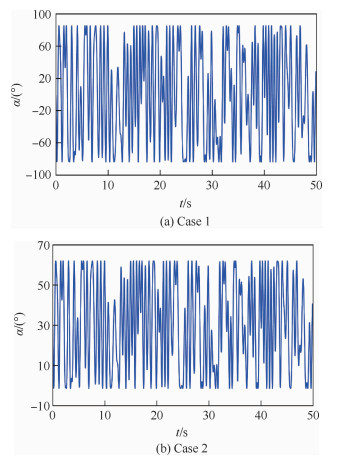图 4 正交相位优化多正弦输入 Fig. 4 Orthogonal phase-optimized multisine input
1.3 训练所用输入形式的选择

 $\mathit{\boldsymbol{x}} = {[\alpha (\tau ),\alpha (\tau - 1), \cdots ,\alpha (\tau - m)]^{\rm{T}}}$ （8）

2 最小二乘支持向量机 2.1 基本理论

 $\left\{ {\begin{array}{*{20}{l}} {\mathop {{\rm{min}}}\limits_{\mathit{\boldsymbol{w}},b,\xi } }&{R = \frac{1}{2}{{\left\| \mathit{\boldsymbol{w}} \right\|}^2} + \frac{1}{2}c\sum\limits_{i = 1}^n {\xi _i^2} }\\ {{\rm{s}}{\rm{.t}}{\rm{.}}}&{{y_i} = {\mathit{\boldsymbol{w}}^{\rm{T}}}\mathit{\boldsymbol{\varphi }}({\mathit{\boldsymbol{x}}_i}) + b + {\xi _i}\;\:i = 1,2, \cdots ,n} \end{array}} \right.$ （9）

 $\begin{array}{*{20}{c}} {L(\mathit{\boldsymbol{w}},b,{\xi _i},{\lambda _i}) = \frac{1}{2}{{\left\| \mathit{\boldsymbol{w}} \right\|}^2} + \frac{1}{2}c\sum\limits_{i = 1}^n {\xi _i^2} - }\\ {\sum\limits_{i = 1}^n {{\lambda _i}} ({\mathit{\boldsymbol{w}}^{\rm{T}}}\mathit{\boldsymbol{\varphi }}({\mathit{\boldsymbol{x}}_i}) + b + {\xi _i} - {y_i})} \end{array}$ （10）

 $\frac{{\partial L}}{{\partial \mathit{\boldsymbol{w}}}} = 0,\frac{{\partial L}}{{\partial b}} = 0,\frac{{\partial L}}{{\partial {\xi _i}}} = 0,\frac{{\partial L}}{{\partial {\lambda _i}}} = 0$ （11）

 ${\mathit{\boldsymbol{w}} = \sum\limits_{i = 1}^n {{\lambda _i}} \mathit{\boldsymbol{\varphi }}({\mathit{\boldsymbol{x}}_i})}$ （12）
 ${\sum\limits_{i = 1}^n {{\lambda _i}} = 0}$ （13）
 ${c{\xi _i} = {\lambda _i}}$ （14）
 ${{y_i} = {\mathit{\boldsymbol{w}}^{\rm{T}}}\mathit{\boldsymbol{\varphi }}({\mathit{\boldsymbol{x}}_i}) + b + {\xi _i}}$ （15）

 ${y_i} = \sum\limits_{l = 1}^n {{\lambda _l}} \langle \mathit{\boldsymbol{\varphi }}({\mathit{\boldsymbol{x}}_i}),\mathit{\boldsymbol{\varphi }}({\mathit{\boldsymbol{x}}_l})\rangle + b + \frac{1}{c}{\lambda _i}$ （16）

 $\left[ {\begin{array}{*{20}{c}} {\mathit{\boldsymbol{K}} + {c^{ - 1}}{{\bf{1}}_n}}&{{{\bf{1}}_n}}\\ {{\bf{1}}_n^{\rm{T}}}&{\bf{0}} \end{array}} \right]\left[ {\begin{array}{*{20}{l}} \lambda \\ b \end{array}} \right] = \left[ {\begin{array}{*{20}{l}} \mathit{\boldsymbol{y}}\\ 0 \end{array}} \right]$ （17）

 $f(\mathit{\boldsymbol{x}}) = \sum\limits_{i = 1}^n {{\lambda _i}} K(\mathit{\boldsymbol{x}},{\mathit{\boldsymbol{x}}_i}) + b$ （18）
2.2 模型参数的确定

SVM通过引入核函数避免了在高维特征空间直接计算内积，极大简化了计算。根据泛函的有关理论，任意满足Mercer条件的对称函数都能作为核函数，最常采用的是高斯核函数：

 $K({\mathit{\boldsymbol{x}}_i},{\mathit{\boldsymbol{x}}_l}) = {\rm{exp}}\left( { - \frac{{{{\left\| {{\mathit{\boldsymbol{x}}_i},{\mathit{\boldsymbol{x}}_l}} \right\|}^2}}}{{2{\sigma ^2}}}} \right)$ （19）

2.3 训练方法

3 训练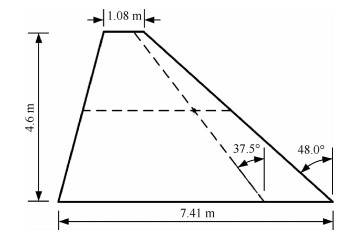图 5 机翼外形图 Fig. 5 Wing shape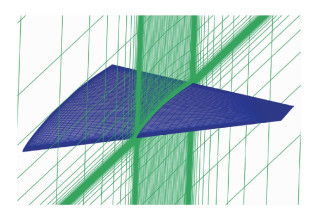图 6 机翼网格生成图 Fig. 6 Sample grid generated around wing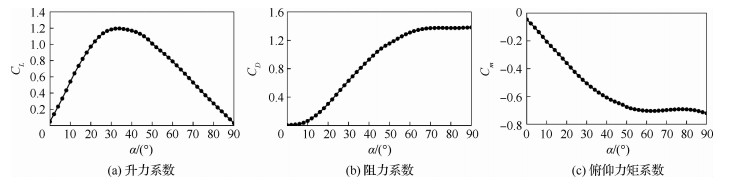图 7 机翼在Ma=0.4、Re=3.0×106下的定常气动力系数 Fig. 7 Steady aerodynamic coefficients of wing at Ma=0.4, Re=3.0×106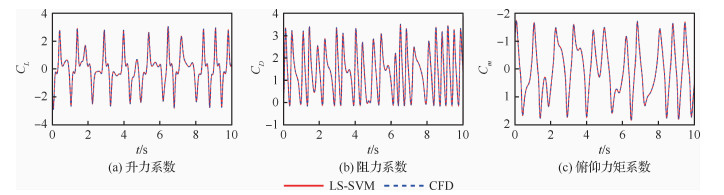图 8 Case 1：初始迎角α0=0°时LS-SVM预测结果与训练数据对比(前10 s) Fig. 8 Case 1: LS-SVM predictions and training data when initial angle of attack α0=0°(first 10 s)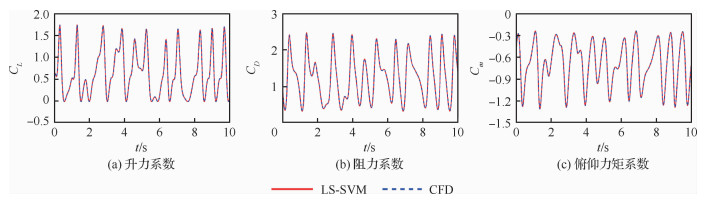图 9 Case 2：初始迎角α0=30°时LS-SVM预测结果与训练数据对比(前10 s) Fig. 9 Case 2: LS-SVM predictions and training data when initial angle of attack α0=30° (first 10 s)
4 非定常气动力验证

4.1 Case 1

Case 1的基准迎角为0°，幅值为80°，机翼做俯仰运动时迎角变化为

 $\left\{ {\begin{array}{*{20}{l}} {\alpha (t) = 80{\rm{sin}}(1.191t)}\\ {\alpha (t) = 80{\rm{sin}}(3.573t)}\\ {\alpha (t) = 80{\rm{sin}}(7.147t)}\\ {\alpha (t) = 80{\rm{sin}}(0.142{t^2})} \end{array}} \right.$ （20）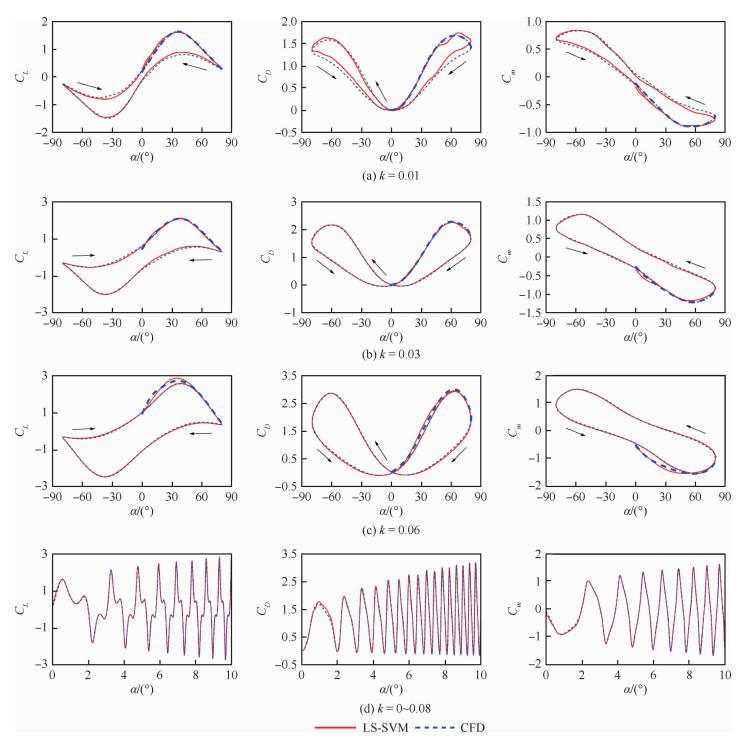图 10 初始迎角α0=0°时LS-SVM预测结果与CFD结果 Fig. 10 Results of LS-SVM predictions and CFD data when initial angle of attack α0=0°

4.2 Case 2

Case 2的基准迎角为30°，幅值为30°，机翼做俯仰运动时迎角变化为

 $\left\{ {\begin{array}{*{20}{l}} {\alpha (t) = 30 + 30{\rm{sin}}(1.191t)}\\ {\alpha (t) = 30 + 30{\rm{sin}}(3.573t)}\\ {\alpha (t) = 30 + 30{\rm{sin}}(7.147t)}\\ {\alpha (t) = 30 + 30{\rm{sin}}(0.142{t^2})} \end{array}} \right.$ （21）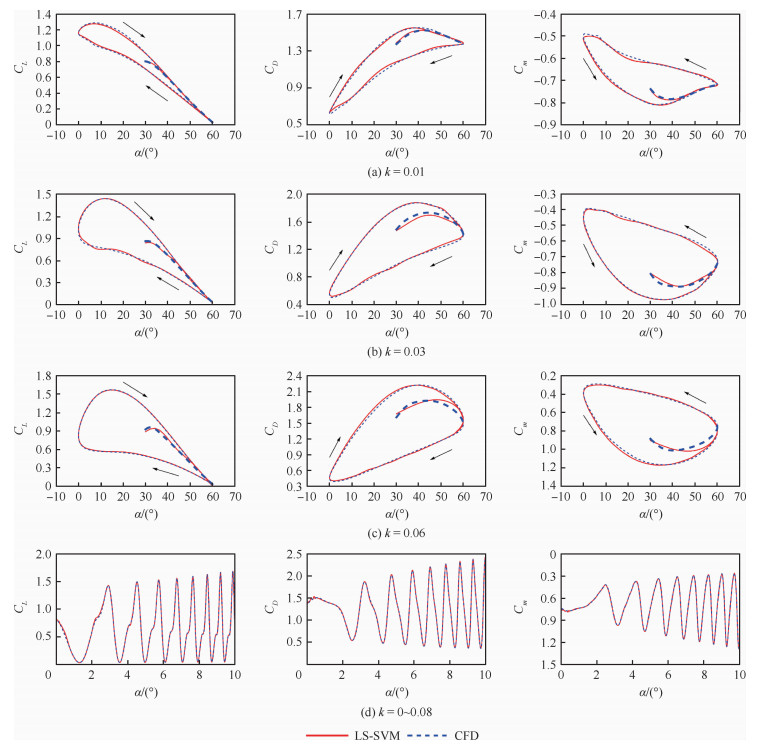图 11 初始迎角α0=30°时LS-SVM预测结果与CFD结果 Fig. 11 Results of LS-SVM predictions and CFD data when initial angle of attack α0=30°

Case 2相比Case 1，基准迎角发生了变化，气动力的初始非稳定过程表现得更为明显，也就是说，在Case 2状态下，气动力的初始段和稳定滞环存在明显差异。根据前面的分析，飞机的真实机动过程始终处于初始段，不会到达稳定滞环。因此可以认为，在当前状态下，仅依靠稳定滞环来预测飞机机动过程中的非定常气动力将存在很大误差。

4.3 以强迫振动为训练输入时的预测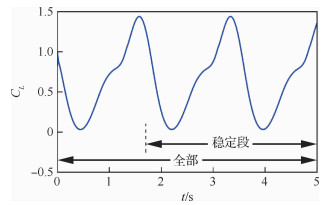图 12 初始迎角α0=30°时由CFD计算的升力系数训练输入(减缩频率k=0.03) Fig. 12 Training data of lift coefficient from CFD with a reduced frequency of k=0.03 when initial angle of attack α0=30°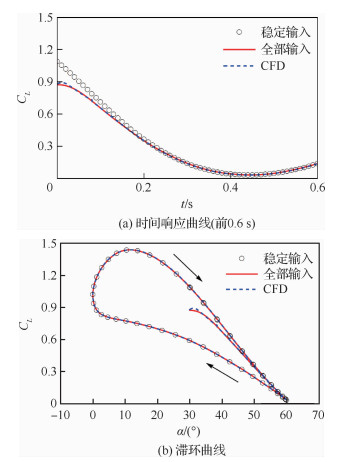图 13 初始迎角α0=30°时不同训练输入下LS-SVM预测结果与CFD结果(减缩频率k=0.03) Fig. 13 Results of LS-SVM predictions and CFD data with a reduced frequency of k=0.03 and different training inputs when initial angle of attack α0=30°

5 结论

1) 基于振动理论分析发现，非定常气动力的动态响应过程存在非稳定和稳定两个阶段，传统非定常气动力建模方法的关注点在稳定阶段，即气动力滞环的建模预测，而飞机的真实机动过程常处于进入滞环的非稳定阶段。

2) 采用最小二乘支持向量机进行大迎角非定常气动力建模，通过对某机翼在大迎角范围内做俯仰运动时升力系数、阻力系数和俯仰力矩系数包含初始非稳定过程在内的全过程准确预测，验证了所用方法对非稳定动态过程非定常气动力的预测能力。

3) 通过选择和设计合理的训练输入以激励系统的非线性特征，代替了常见的以多个强迫振动试验数据为输入，简化了训练过程，避免了以滞环预测滞环。在输入的形式选择上考虑到气动力对运动过程的依赖，舍弃了常见的以减缩频率为参数，建立了不依赖于频率的能反映任意运动过程的具有普遍意义的气动力模型。

4) 开始运动的基准状态对气动力的非稳定动态过程存在明显影响。在某些情况下，初始非稳定过程会和稳定滞环重合，即差异表现不明显，但在其他情况下，初始非稳定过程会和稳定滞环存在显著区别。

http://dx.doi.org/10.7527/S1000-6893.2020.23675

0

#### 文章信息

CHEN Senlin, GAO Zhenghong, ZHU Xinqi, PANG Chao, DU Yiming, CHEN Shusheng

Unsteady aerodynamic modeling of unstable dynamic process

Acta Aeronautica et Astronautica Sinica, 2020, 41(8): 123675.
http://dx.doi.org/10.7527/S1000-6893.2020.23675